# 3.学习内容

• ## 算法效率衡量

• ### 大O记法

• 时间复杂度：假设存在函数f，使得算法A处理规模为n的问题示例所用时间为T(n)=O(f(n))，则称O(f(n))为算法A的渐近（忽略常数）时间复杂度，简称时间复杂度，记为T(n)

• 最优时间复杂度
• 最坏时间复杂度
• 平均时间复杂度
• ### 时间复杂度的几条基本计算规则

• 一条基本语句 时间复杂度为 O(1)
• 顺序结构，时间复杂度用加法
• 循环结构，时间用乘法
• 分支结构，时间复杂度取最大值（for n次里面 if n,就是n^2）
• 判断一个算法的效率，关注操作数量最多的，其余次要项和常数项省略
• 没有特殊说明，算法时间复杂度说的一定是最坏时间复杂度
• ### 常见时间复杂度

• 执行次数函数举例 非正式术语
12 O(1) 常数阶
2n+3 O(n) 线性阶
3n2+2n+1 O(n2) 平方阶
5log2n+20 O(logn) 对数阶
2n+3nlog2n+19 O(nlogn) nlogn阶
6n3+2n2+3n+4 O(n3) 立方阶
2n O(2n) 指数阶
• 注意，经常将log2n（以2为底的对数）简写成logn
• ## 常见时间复杂度之间的关系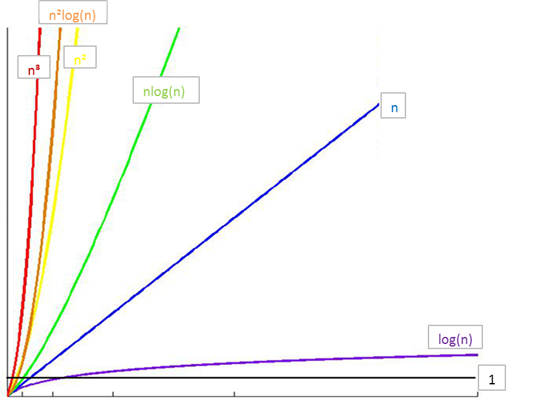• 所消耗的时间从小到大
• O(1) < O(logn) < O(n) < O(nlogn) < O(n2) <O(n^2logn)< O(n3) < O(2n) < O(n!) < O(nn)
• ## Python中List内置操作时间复杂度

• python的extend方法比+的效率要高
• 尽量不要用+来操作列表，+=倒是可以
• +=是被优化过的，所以跟extend差不多
•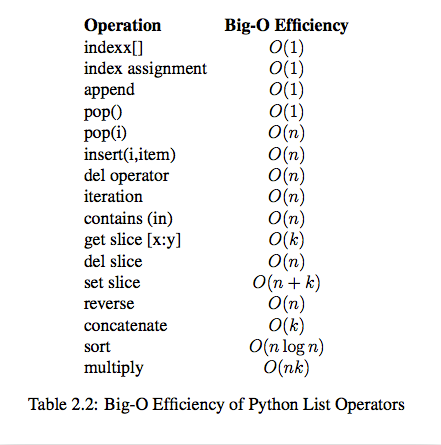• ## Dict内置操作时间复杂度时间复杂度

•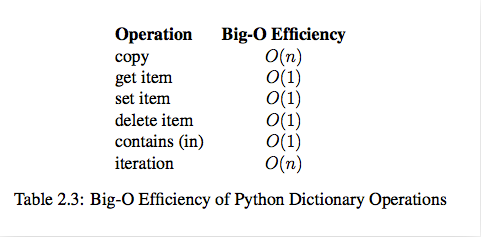• ## 数据结构

• ### 数据结构定义

• 一些数据（int float str）按照一定结构 (name,age,score)组成的关系，就是数据结构
• ### python的内置数据结构

• 列表 ，元组，字典
• 自定义扩展数据结构
• ### 算法与数据结构

• 程序=数据结构+算法
• 算法是为了解决实际问题而设计的，数据结构是算法需要处理的数据载体
• ### 抽象数据类型(Abstract Data Type)

• 常用的数据运算：插入、删除、修改、查找、排序
• ### 线性表

• #### 顺序表，就是地址是连续的，列表就是顺序表的实现

• ##### 顺序表的基本布局（传统）：
• 索引之所以从零开始，就是因为没有地址偏移量，
• 比如存放int数据，int占用四字节，所以第二个元素就是从相对上一个元素，偏移1个单位（int四个字节）
• 顺序表的构成，
• 开头是容量和个数
•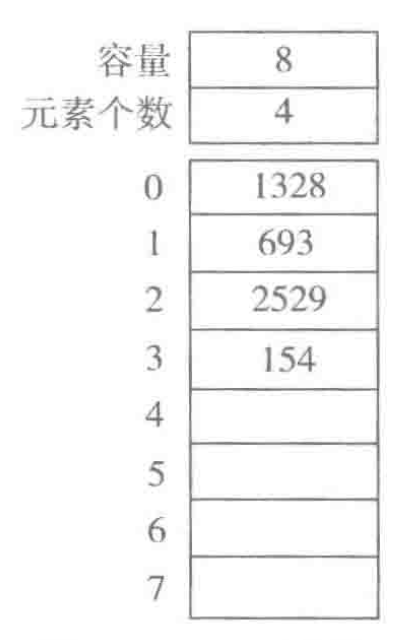• 顺序表有两种实现方法
• 一体式结构
• 地址连续，从头到尾
• 分离式结构
• 一个头指针存放容量，个数，还有一个指向列表的指针
• 元素存储区替换(一体式结构)
• 线性表扩充时候，是所有元素搬迁，全部移动到新开辟的数组中
• 元素存储区扩充（分离式结构）
• 推荐按照数量扩充，以空间换时间
• 每次扩充，有两种方式，一种是按数量扩充，一种是按倍数扩充
• 按数量特点：
• 节省空间，但是扩充操作频繁，操作次数多
• 按倍数特点：
• 减少了扩充操作的执行次数，但可能会浪费空间资源
• ##### 元素外置顺序表（存地址）
•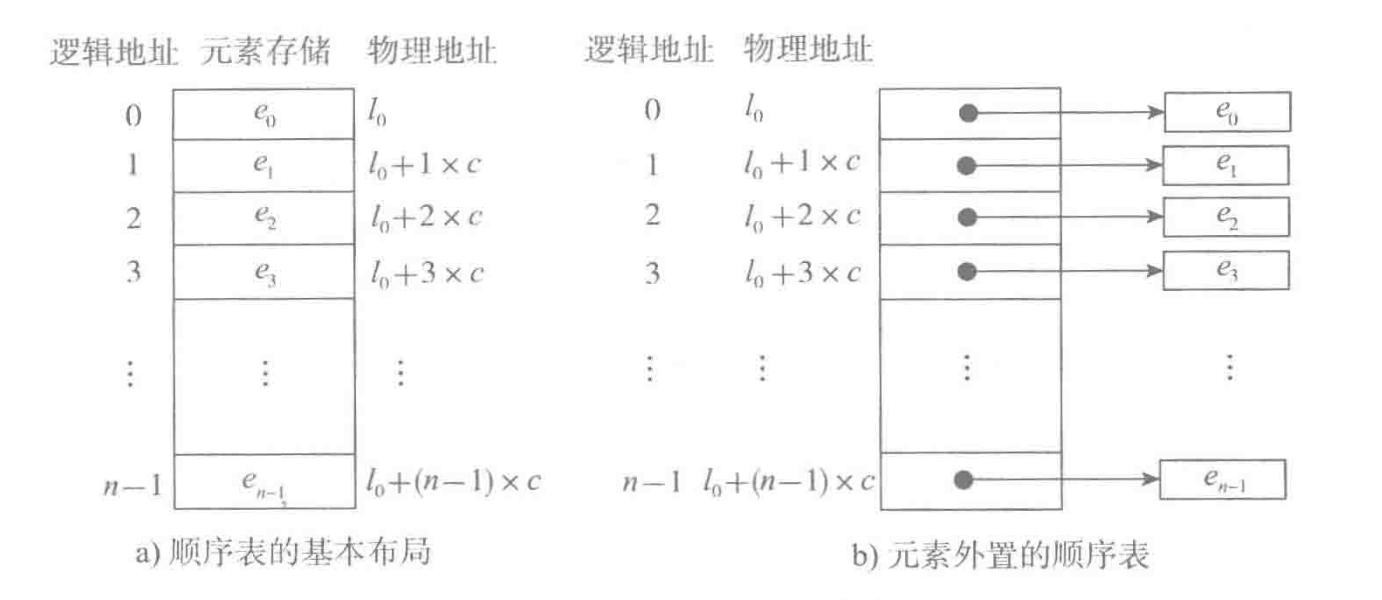# 4.扩展延伸知识

• ## python的变量本质

• ### python单双下划线

• "单下划线" 开始的成员变量叫做保护变量，类对象和子类对象可以访问；
• "双下划线" 开始的是私有成员，只有类对象可以访问
• _xxx 不能用'from moduleimport *'导入
• __xxx__ 系统定义名字
• __xxx 类中的私有变量名

Ares个人进阶之路